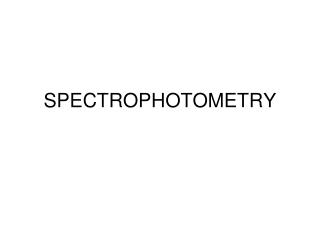DownloadDownload PresentationSPECTROPHOTOMETRY

SPECTROPHOTOMETRY

Télécharger la présentationSPECTROPHOTOMETRY

- - - - - - - - - - - - - - - - - - - - - - - - - - - E N D - - - - - - - - - - - - - - - - - - - - - - - - - - -
Presentation Transcript

1. SPECTROPHOTOMETRY

2. Spectrophotometry • Determines concentration of a substance in solution • Measures light absorbed by solution at a specific wavelength

3. Spectrophotometry • One of the simplest and most widely used methods to determine the amount of protein or nucleic acid present in a given solution

4. Spectrophotometry • Proteins do not absorb in visible wavelength region unless they have a prosthetic group (e.g., Fe2+), or an unnatural amino acid

5. Spectrophotometry • Visible region: low energy electronic transition due to: a. Compounds containing transition metals b. Large aromatic structures & conjugated double bond systems (vitamin A, heme) • UV region (200-400 nm): a. Small conjugated ring systems (Phe, Tyr, Trp)

6. Spectrophotometry Io I A = 0.012  l  Lamp Monochromator Detector Cuvette

7. Spectrophotometers • Light source (Lamp) • Optical filters or prism • Tube or cuvette • Photocell or photomultiplier tube

8. Light source (Lamp) • Visible region = tungsten or tungsten-halogen • UV light = deuterium or hydrogen lamp

9. Optical filters/prisms • To limit light to a certain wavelength • Monochromator can isolate a specific wavelength of white light and allow it to pass through the solution being analyzed

10. Tubes or cuvettes • Visible range = glass cuvette • UV range = quartz cuvette

11. Photocell • To detect transmitted light

12. Spectrophotometry • Beer-Lambert’s Law log Io = cl I Where: Io = intensity of incident light I = intensity of transmitted light  = molar extinction coefficient c = concentration of the absorbing species (mol/L) l = path length of the light-absorbing sample (cm)

13. Beer-Lambert’s Law • The fraction of the incident light absorbed by a solution at a given wavelength is related to a. thickness of the absorbing layer (path length) and b. concentration of the absorbing species

14. Visible region wavelength

15. Beer-Lambert’s Law • Concentration  amount of light absorbed A = abc = log(100/%T) Where A = absorbance a = absorptivity of the compound under standard conditions b = light path of the solution c = concentration of the compound %T = percent transmittance

16. Beer-Lambert’s Law • Absorbance A = K x C = Log10Io I Where: Io = amount of light absorbed by the solution expressed as absorbance or optical density K = constant C = concentration of the substance

17. Transmittance • Defined as the ratio of the intensity of light emerging from the solution (I) to that of incident light entering (Io) T = I Io Io I

18. Transmittance • Inversely related to the concentration of the solution and is expressed in % % T = 1 x 100 Io

19. Transmittance • 100% transmittance means no light is absorbed by the solution so that incident light is 100% transmitted

20. Absorbance & Transmittance • Absorbance  concentration • Transmittance 1/  to concentration and absorbance

21. Sample Problem • Cytosine has a molar extinction coefficient of 6 x 103 mol-1 cm-1 at 270 nm at pH 7. Calculate absorbance of 1 x 10-3 M cytosine solution in 1mm cell at 270 nm A = Log I0= lc I

22. Sample Problem • Solution: 1. A = lc = (6 x 103)x (0.1) x (1 x 10-3) = 6 x 10-1 = 0.6 (O.D.)

23. Spectrophotometry • Clinical applications: 1. Aromatic amino acids have characteristic strong absorbance of light at a wavelength of 280nm ex. Tryptophan & tyrosine

24. Calibration Curve